Main Body

# Introduction and Units

Units

### Learning Objectives

At the end of this introductory chapter you should be able to

• Demonstrate familiarity with the study procedure, performance expectations and components of the course
• Perform units conversions problems within SI and US Customary systems
Study procedure

Delivery of this course is based on the Applied Strength of Materials for Engineering Technology, by Dr. Barry Dupen.  This resource will be referred to as the “textbook“.

To complement the textbook students have access to the current resource, further identified as “supplement“.  This consists of summaries of main concepts developed in the textbook and assigned problems.

For best results students should adhere to the following sequence:

1. Before class, study the theory and review the sample problems in the textbook. Some topics were already covered in Applied Physics but you will benefit from a brief review.
2. To reinforce the concepts, review the key notes in the supplement.  Take notes of the concepts you found challenging and ask for clarifications in class.
3. Classroom lectures:
•     Instructor will review the theoretical concepts and answer questions
•     Instructor will demonstrate solving selected problems.  When needed, instructor’s notes will be published on line.
•     Students will solve assigned problems in small groups, with guidance from instructor
4. Individual work
•     Students will solve  assigned problems on their own, for self-evaluation.
•     Instructor will provide guidance and feedback during posted office hours or Tutorial Sessions

Course evaluation:

• Each chapter will be assessed through quizzes or assignments.
• Midterm and Final examinations.  Combined passing score is 60%.
• Attendance will be monitored but is not mandatory.
Recommendations

Strength of Materials is a “methodical” discipline.  This means that it deals in general with standard/classical questions that usually have an established method of solving them.  When solving problems students often follow steps and procedures that were previously demonstrated in class or in the textbook.  These approaches are logical and never students would be expected to memorize them.  However, it is important for students to practice solving questions on their own since this will help them see patterns in questions, provide them with problems solving experience and help them complete the exercise in the allotted time.

For best results, students are encouraged to work after classes between 2 and 3 hours for each hour of lecture.  This effort will be different for each student.  To manage your time more efficiently consider attending the weekly scheduled tutorials.

Units and conversions

Like in many other engineering disciplines calculations may be performed in both systems of units, US Customary and SI.  While Canada has officially adopted the SI (metric) system in 1970, economic cooperation with US companies requires engineering graduates to be fluent in both systems.  Some computational software that you will use may be available only in US Customary units, being developed in US, and mostly for American users.  It is therefore imperative to be able to complete calculations in both systems of units and to be able to convert between systems.

When solving problems, if the data is given in SI units, complete the solution in SI units.  Similarly for US Customary units; there is not need to switch the system of units.

In the metric system prefixes are added to base and derived units to form names and symbols that are multiples of SI units. The following table shows the commonly used SI prefixes.

 Prefix Symbol Multiplying Factor Giga X GX 109 = 1000 000 000 Mega X MX 106 = 1000 000 Kilo X kX 103 = 1000 Hecto X hX 102 = 100 Deca X daX 101 = 10 Base SI Unit “X” “X” can be m, g, W, J, etc. 100 = 1 Deci dX 10-1 = 0.1 Centi cX 10-2 = 0.01 Milli mX 10-3 = 0.001 Micro μX 10-6 = 0.000 001

There are different ways to perform units conversions but in the end, they all lead to the same answer.  The following are simple examples to demonstrate the procedure.

Examples – SI system:

1.  Convert 0.2 km to cm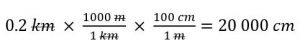• When performing SI conversions it is easy to see if your answer is reasonable or not.  For instance if you move from a large unit (kilo) to a smaller one (centi), the resulting value should be greater.
• Looking at Fig. 1, you may also consider moving the decimal point to the right, three steps from Kilo to base and two more steps from base to your final answer.  This is an alternative approach to performing SI conversions.

2.  Convert 50 000 cW to kW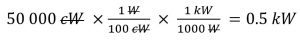• Note that some units may be presented with a less commonly used prefixes.  For instance, while “centimeter” is frequently used, “centiwatts” not so much.  However, you should be able to identify the prefix and the unit it applies to.

3.  Convert 300 000 cm3 to dam3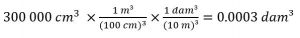•   You may look at this conversion as follows: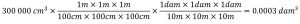• Pay extra attention when using powers, as in volume or area conversions.

For the purpose of this course most of the US Customary conversions will deal with linear dimensions.  The conversion factors we use are presented in Appendix A.  It is desirable to remember the most used factors such as 1 ft = 12 in or 1 yd = 3 ft.

Examples – US Customary system

4.   Convert 1.2 yards to inches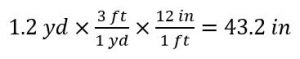5.   Convert 2 square feet to square inches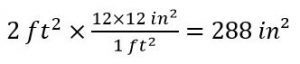### Assigned Problems

Problem 1: The hoop stress in a pressure vessel is calculated with the formula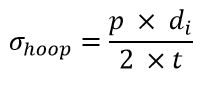where p is the design pressure, di is the inside diameter and t is the wall thickness.

1. If p = 4450 kPa, di = 1.8 m and t = 20 mm, determine the hoop stress in the wall, in MPa.
2. If p = 645 psi, di = 6 feet and t = ¾ in,  determine the hoop stress in the wall, in ksi.

Problem 2: To determine the dead load on a foundation you are required to estimate the weigh of a spherical tank (V=4/3 πr3), full with a liquid of given density.  Tank mass is negligible compared to the mass of the product.  Determine its weight based on the following:

1. Diameter = 200 cm, density = 1.12 g/cm3.  Answer in N.
2. Diameter = 80 in., density = 70 lb/ft3.  Answer in lb.

Problem 3: Suggest one improvement to this chapter.

The improvements have to be specific and clear, for example:

• correct this typo
• replace this phrase with this
• add this explanation to this section
• add this problem to the chapter problems
• etc

You may use screen captures to identify the section that you would like improved or expanded.